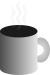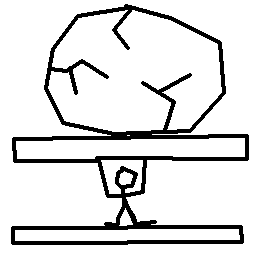#Coffee SpaceListen:

## Failed CompressionPreview Image

### Introduction

A while back, I was working on a small compression algorithm for high entropy data, specifically for a tiny hobby operating system back in 2017. The idea was that I would use 256 bytes or less for decompression code that would decompress an operating system hiding in a 512 byte boot sector.

Unfortunately the project didn't work out, but a recent post on the Pine forum got me thinking. Specifically:

This file is then lz4 compressed, and written to the spi flash of the pinetime. The pinetime decompresses, processes the blocks, and writes them to the display.

That sounds awesome :) Do you get much savings with lz4? I assume the data you are sending is already pretty high entropy?

It goes from 5046208 bytes to 4099181. Which makes it small enough to fit in the 4 MiB of spi flash

This lz4 lossless compression algorithm sounds awesome. It made me think about my previous attempts and what went wrong.

### Compression Attempts

The following are some failed methods I created to try and compress high-entropy data. I thought about these quite some years ago, but it's interesting to look back on them and see so obviously now why they couldn't possibly work 1.

#### Grid Compression

The idea was pretty simple with this one - you take a matrix and then attempt to create some abstract representation of it by performing a calculation.

Defining it better, we can give some example matrix of values $a$, $b$, $c$ and $d$:

$\mid \begin{array}{cc}a& b\\ c& d\end{array}\mid$

We can then sum each of the rows and columns to 'compress' the data: $\left[$ $a+b$, $c+d$, $a+c$, $b+d$ $\right]$. To recover the data, you simple need to solve the matrix and satisfy the summed values. This could be done trivially and the data is always perfectly recoverable.

Of course, there are no real savings here - you store four values as four different values. The area is ${n}^{2}$ and the summed data is $2n$. But we see the possibility of savings when we expand the matrix, for $n=3$ we in theory can compress 9 bytes down to 6 bytes:

$\mid \begin{array}{ccc}a& b& c\\ d& e& f\\ g& h& i\end{array}\mid$

And the data would be compressed like so: $\left[$ $a+b+c$, $d+e+f$, $g+h+i$, $a+d+g$, $b+e+h$, $c+f+i$ $\right]$. But this should be setting your bullshit sensors off - we can simply apply this to any arbitrary data any arbitrary number of times. Something is seriously wrong here...

• Compression count - Firstly, you would need a value to contain the number of times the operation was performed in order to undo it.
• Multiple solutions - Of course, by far the largest problem is that the $n=3$ matrix has multiple solutions. The only way to solve this problem is to introduce another column in the third dimension in order to break symmetry. This of course adds an additional 3 bytes and leads to no savings. This also appears to be true for all cases where $n\ge 3$.

#### Bubble Sort Compression

The idea essentially was to implement a reversible bubble sort algorithm - each sort the algorithm does it leaves a symbol in place to indicate that a sort occurred. Eventually the idea is that all of the symbols would be in order, and then a table simply needs to store the frequencies of each character.

Essentially we had some string we wanted to sort, and a special symbol $z$ - where $z$ had some magic value that placed it in the middle of the data when fully sorted. If we use the string $\left[$ $a$, $b$, $c$ $\right]$, we would initially check if $a>b$. If so, we need to swap them. The string would then become $\left[$ $b$, $z$, $a$, $c$ $\right]$. You would then repeat this until the sorting is completed, and store the index of the last location to be sorted.

To decompress, you then reverse this operation, so $\left[$ $b$, $z$, $a$, $c$ $\right]$ becomes $\left[$ $a$, $b$, $c$ $\right]$, where the $z$ is removed after reversing the operation.

I implemented this and of course, it's not perfect 2. The main issues with the algorithm are:

• Disk requirements - The bubble sort method uses so many characters (over so many sort operations) that the size of the frequency data ends up being the same size or larger than the original data you were trying to compress.
• RAM requirements - Even expanding the frequency data would be nowhere near to possible on modern hardware, let alone in 1MB 16 bit mode. I estimated back then that for 512 bytes, you would need $1×{10}^{167}$ bytes of RAM.
• Compression - Compression time was "reasonable" (compared to everything else), but still also grew exponentially. Compressing 512 bytes would take near to a lifetime to complete.
• Decompression - Fortunately, decompression time was much quicker - but still suffered from an exponential curve. For 512 bytes, you couldn't reasonably hope for a computer to finish in your lifetime.

The original article has more details on the issues involved, but it clearly is not so great.

### Future Compression

So, how can I proceed with more viable compression methods?

#### LZ4

Of course this is a very decent compression algorithm, which is extremely fast and well supported. But it's not particularly suitable for this application for multiple reasons:

1. Large data - It only appears to perform well on large blocks of data. When I was testing on ~2kB ASCII data I saw no compression improvement, often actually delivering a worse result.
2. Implementation - There is a surprising amount going on - you really do not want to waste so much code space encoding the problem at this level.

#### Custom Huffman Coding

I believe I will attempt to incorporate something like Huffman coding as it is very simple. Some characters in the binary are worth representing in smaller coding forms (such as a NULL byte or a `jmp` instruction), whilst many characters either not represented or appear only once or twice in a single block (512 bytes).

Given that only a small subset of bytes can benefit from the coding process, it therefore makes sense to not build a full Huffman coding tree for all 256 symbols but instead only bother building a tree for the most represented data (say the first 16 or 32 bytes). After this, all remaining unrepresented bytes can be assumed to be encoded in order 3.

### Future

Why keep on working on this? Well, I still like the OS project of course - but also a compression algorithm that can work on arbitrarily small blocks of data without inter-lock dependency could also be extremely useful. One example is in the audio space, where Google are testing audio streams below 3kbps. I have also seen audio all the way down to 450bps. Compression that can then be applied on top of this could make a big difference.

One application I have been thinking about in the back of my mind is LoRa communications - at some point I really want to revisit my LoRa-chan project and get some form of audio streamed without absolutely demolishing the network.

1. If we can look back on our past selves and find improvement, this is a great sign of progress.

2. I believe that initial implementation was wrong, but the idea is so ridiculous that I don't want to invest time into fixing it.

3. This will of course require some thinking for optimal implementation